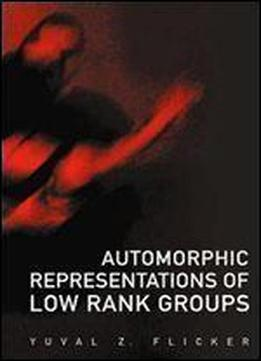# Automorphic Representations Of Low Rank GroupsTags: Yuval Z. Flicker

The area of automorphic representations is a natural continuation of studies in number theory and modular forms. A guiding principle is a reciprocity law relating the infinite dimensional automorphic representations with finite dimensional Galois representations. Simple relations on the Galois side reflect deep relations on the automorphic side, called "liftings". This book concentrates on two initial examples: the symmetric square lifting from SL(2) to PGL(3), reflecting the 3-dimensional representation of PGL(2) in SL(3) and basechange from the unitary group U(3, E/F) to GL(3, E), [E : F] = 2. The book develops the technique of comparison of twisted and stabilized trace formulae and considers the "Fundamental Lemma" on orbital integrals of spherical functions. Comparison of trace formulae is simplified using "regular" functions and the "lifting" is stated and proved by means of character relations. This permits an intrinsic definition of partition of the automorphic representations of SL(2) into packets, and a definition of packets for U(3), a proof of multiplicity one theorem and rigidity theorem for SL(2) and for U(3), a determination of the self-contragredient representations of PGL(3) and those on GL(3, E) fixed by transpose-inverse-bar. In particular, the multiplicity one theorem is new and recent. There are applications to construction of Galois representations by explicit decomposition of the cohomology of Shimura varieties of U(3) using Deligne's (proven) conjecture on the fixed point formula.